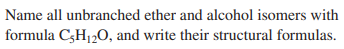×
Get Full Access to Fundamentals Of General, Organic, And Biological Chemistry (Mastering Chemistry) - 8 Edition - Chapter 14 - Problem 14.60
Get Full Access to Fundamentals Of General, Organic, And Biological Chemistry (Mastering Chemistry) - 8 Edition - Chapter 14 - Problem 14.60

×ISBN: 9780134015187 2044

## Solution for problem 14.60 Chapter 14

Fundamentals of General, Organic, and Biological Chemistry (Mastering Chemistry) | 8th Edition

• Textbook Solutions
• 2901 Step-by-step solutions solved by professors and subject experts
• Get 24/7 help from StudySoup virtual teaching assistantsFundamentals of General, Organic, and Biological Chemistry (Mastering Chemistry) | 8th Edition

4 5 1 389 Reviews
23
0
Problem 14.60

Name all unbranched ether and alcohol isomers with formula $$\mathrm{C}_{5} \mathrm{H}_{12} \mathrm{O}$$ , and write their structural formulas.

Text Transcription:

C_5H_12_OStep-by-Step Solution:
Step 1 of 3

Step 2 of 3

Step 3 of 3

## Discover and learn what students are asking

Calculus: Early Transcendental Functions : Product and Quotient Rules and Higher-Order Derivatives
?Using the Product Rule In Exercises 1–6, use the Product Rule to find the derivative of the function. $$f(x)=e^{x} \cos x$$

Calculus: Early Transcendental Functions : Second-Order Nonhomogeneous Linear Equations
?Verifying a Solution In Exercises 1-4,verify the solution of the differential equation. Solution D

Statistics: Informed Decisions Using Data : Applications of the Normal Distribution
?In Problems 19–22, find the value of ?? ?0.01

Statistics: Informed Decisions Using Data : Comparing Three or More Means (One-Way Analysis of Variance)
?Car-Buying Discrimination To determine if there is gender and/or race discrimination in car buying, Ian Ayres put together a team of fifteen white mal

Unlock Textbook Solution